# Verhulst Function for Modeling Minerals Depletion and Product Manufacture

L. David Roper
http://www.roperld.com/personal/RoperLDavid.htm
27 June, 2020

## Minerals Depletion

The Verhulst Function is a good function to use for fitting minerals-depletion data. It is used to model the fact that their is usually an exponential growth in the rate of extraction a mineral from the Earth, followed by a peak, after which the extraction rate declines exponentially. The function is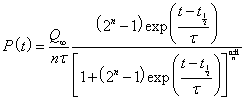..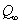is the amount to be eventually extracted,is the rising exponential time constant,is the falling exponential time constant and t1/2 is the time at which the resource is one-half depleted. The parameter n determines the amount of skewing at large times. For n = 1 the extraction curve is symmetrical and the peak occurs at t1/2. The deviation of the peak time from t1/2 is negative for n > 1 (skewed toward large times) and is positive for n < 1 (skewed toward small times).

The maximum of P(t) occurs at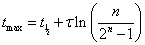, which yields- Note that, for the symmetric case (n=1):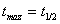and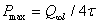.

When a peak is symmetrical, the Verhulst function simplifies to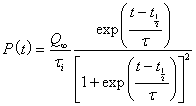.

The asymmetry parameter, n, must be greater than 0. For the case of n=0, the Verhulst function becomes the Gompertz function: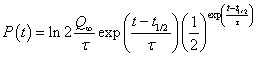.

## Amount Left to be Extracted

The amount left to be extracted at time t is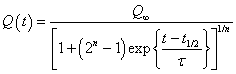.

The amount already extracted is.

This function reduces to the hyperbolic-tangent function when n = 1:## Graphs

The following graph shows the Verhulst function for= 100, t1/2 = 1950 and= 5 with 6 different values of n:The area under all the curves is= 100.

For a symmetric Verhulst function used to fit data up to a time t and given the definitions A = amount already extracted and R = true reserves = amount left to be extracted: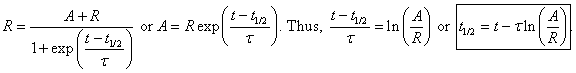.

For a known A, t and, the peak position, t1/2, varies logaithmically with 1/R, which is much less than linearly. If enough data are present in the extration exponential rise, the rate,, can be determined by fitting those data by the Verhulst function. A can be determined by adding up the extraction for all years up to year t.

The following graph shows the amount-left Verhulst function for= 100, t1/2 = 1950 and= 5 with 6 different values of n: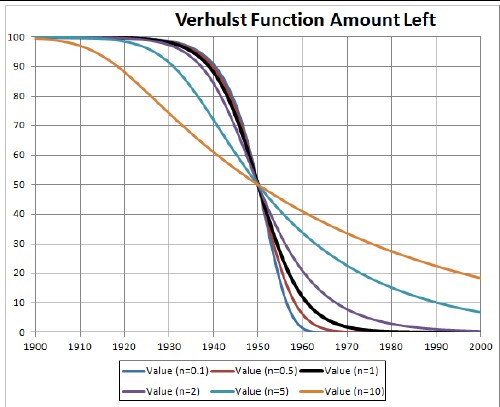Of course, the amount already extracted at time t is-Q(t).

## Duration of Extraction

It is useful to define a "duration" for the extraction of a mineral by the difference in the times when (f-1)/f of it has been extracted and when 1/f of it has been extracted: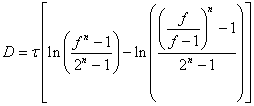.

This is derived from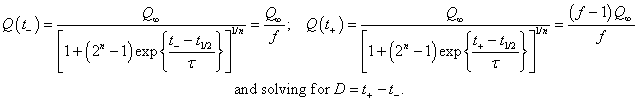.

For the symmetric case (n = 1).

A good choice for f is 10; then the duration would be the time interval for extracting the middle 80% of.

## Fast Decline

The Verhulst function has a limit as to how fast extraction can decline. See the curve for n=0.1 above; putting in parameter n much closer to zero does not change that curve very much. However, the Verhulst function appears to work quite well for minerals extraction; steeper extraction appears to be unlikely. Of course, common bankruptcies of all companies extracting a rare mineral could cause a very steep extraction decline.

Another exponential function that allows much faster decline is: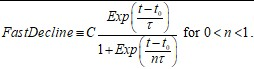This gives a peaked function only for n < 1.

Here is an example curve for n = 0.09 (The declining exponential time constant 0.09 times smaller than the rising time constant.):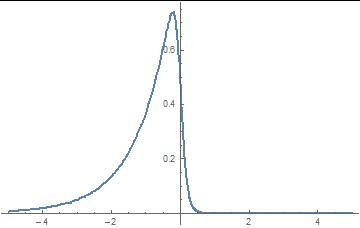Values of n closer to 0 yield a very steep decline, approaching a zero time constant.

To get the cumulative extraction for a mineral, one needs the integration of the extraction function. Unfortunately, the integration of this Fast-Deline function yields the complicated Hypergeometric function: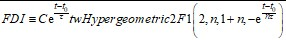Math programs, such as Mathematica, Excel and Scientific Workplace have this function.

Here is an example cumulative curve for n = 0.09: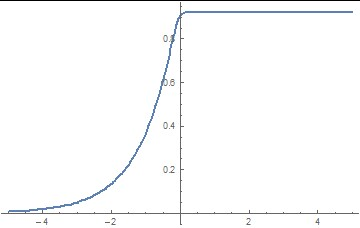## Examples

### Coal Extraction in United Kingdom=32422 x 106 tons, t1/2 = 1913.5,= 47.50 and n = 0.1 .

### Coal Extraction in Virginia USA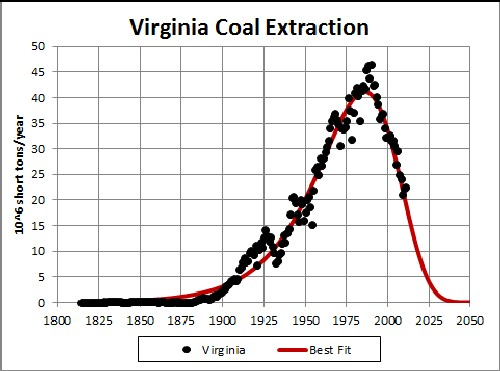=2720 x 106 tons, t1/2 = 1977.3,= 24.22 and n = 0.1 .

### Crude-Oil Extraction in United KingdomFirst peak:=13.52 x 109 barrels, t1/2 = 1985.9,= 2.645 and n = 2.316 .
Second peak:=17.07 x 106 tons, t1/2 = 2004.6,= 1.876 and n = 7.565 .

Back to the top

## Product Manufacture

When a new product is introduced in a factory it often starts out at a low level, then later rises to new levels at time goes on. One could represent this moving from one level to another by a series of hyperbolic tangents transiting from one level to an asymptotic next level.. However, the rising and the asymptotic exponential time constants are the same for a hyperbolic tangent. The Verhulst funtion can have different rising and the asymptotic exponential time constants (and).

The equation for moving from one manufacturing level, QL, to another, QH, is.

The cumulative production is just the summation of the periodic production data.

If there are many levels the production equation is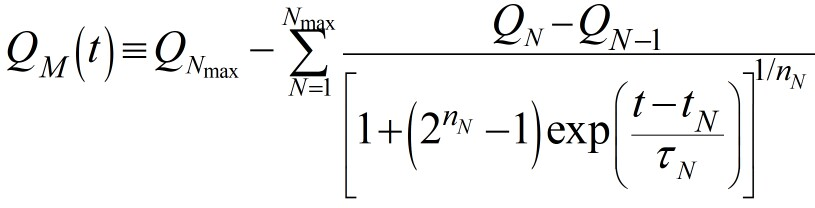, starting at N=0 to N=Nmax.

If the periodic production data are highly uncertain it would be easier to use the hyperbolic-tangent function to fit the levels' data. The rising and the asymptotic exponential time constants are the same for the hyperbolic tangent.

Minerals Depletion

L. David Roper interdisciplinary studies

L. David Roper, roperld@vt.edu
26 March, 2019